## The electrolysis of water has the equation 2H2O (l) → 2H2 (g) + O2 (g). What type of reaction is this? Question 9 options:

Question

The electrolysis of water has the equation 2H2O (l) → 2H2 (g) + O2 (g). What type of reaction is this?
Question 9 options:

A)

Single displacement

B)

Double displacement

C)

Decomposition

D)

Synthesis

in progress 0
2 months 2021-07-22T06:50:28+00:00 1 Answers 3 views 0

1. Answer: The correct option is C) decomposition

Explanation:

Single displacement reaction is defined as the reaction where a more reactive element displaces a less reactive element from its salt solution.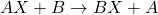Element B is more reactive than element A

Double displacement reaction is defined as the reaction where an exchange of ions takes place in a solution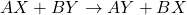Decomposition reaction is defined as the reaction where a single large chemical species breaks down into two or more smaller chemical species.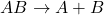Synthesis reaction is defined as the reaction where two or more smaller chemical species combine together in their elemental state to form a single large chemical species.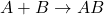For the given chemical reaction: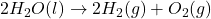Here, water is breaking down into two smaller species of oxygen and hydrogen gas. Thus, it is a decomposition reaction.

Hence, the correct option is C) decomposition